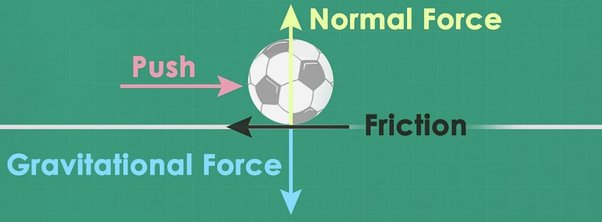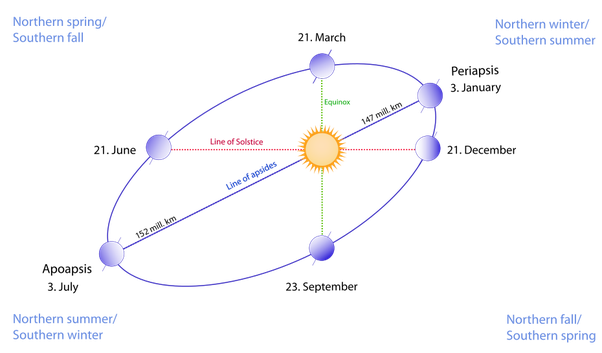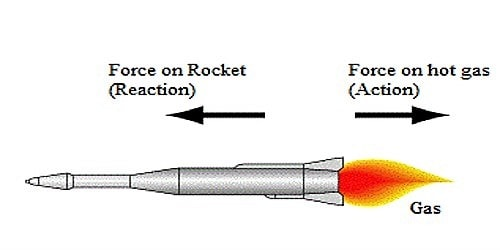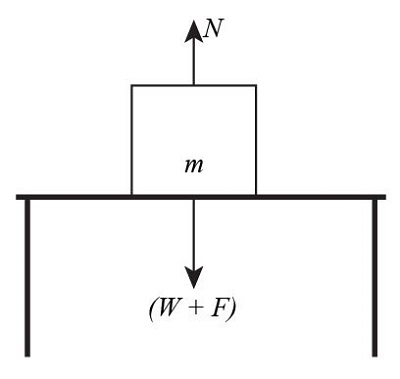# Force and Motion

• Astrophysics• Electricity• Electromagnetism• Energy• Fields• Force• Mechanics• Momentum• Nuclear Physics• Quantities & Units• States of Matter• Waves• Key ExperimentsWhen a football is kicked, it goes flying through the air. This happens because of something called force. Force is what decides how things move. To figure out where an object will go, we need to understand how force and motion are related Sir Isaac Newton was the first person to notice this, and he came up with three laws to explain it. These laws are so good that we can use them to calculate how things move, even when we're walking on the moon!

The first law explains why objects can't move by themselves. The second law helps us figure out how things like cars and rockets move. The third law tells us why guns move backward after they're fired, and how rockets are able to go up.

By understanding these laws, we can explain a lot of the things we see in the world around us. For example, we can see why things fall down, how cars move, and even how rockets get into space. So next time you're watching a football game or looking up at the stars, remember that it's all because of force and motion!

## Forces and motion: Definition

The first page is a landing page. The second page is a blog/article. The third page is a blog/article. The fourth page is a blog/article. The fifth page is a blog/article. The sixth page is a blog/article. The seventh page is a blog/article. It looks like there are more blogs/articles than landing pages, so you should write a blog.

## Forces and motion properties

Forces and Motion: Understanding Vectors

It's important to keep in mind that both velocity and forces are vectors. This means that we need to know their magnitude and direction to define them accurately.

Let's consider an example to help us understand the of the vector nature of velocity in defining an object's state of motion. Imagine a car moving west at a constant speed. After an hour, the car turns and starts moving north at the same speed. Although the car's speed remains the same throughout, its state motion changes, as it starts moving in a different direction.

Similarly, a force is also a vector quantity. It doesn't make sense to talk about forces and motion if we don't specify their magnitude and direction. Before we go into more detail about this, let's talk about the units of force. The SI unit of force is the newton. One newton is the amount of force required to produce an acceleration of one meter per second squared in an object with a mass of one kilogram. Forces are usually represented by the symbol F.

An object can have many forces acting on it, so it's important to know how to deal with multiple forces. In the next section, we'll explore the basics of dealing with multiple forces and how they affect an object's motion.

## Force and motion basics

Understanding Multiple Forces and Their Effect on Motion

Forces play a crucial role in determining the motion of objects. To predict the motion of an object accurately, it's essential to know how to deal with multiple forces acting on it. Since forces are vector quantities, we can add them together by considering their magnitudes and directions.

The sum of a group of forces acting on an object is called the resultant or net force. The resultant force is a single force that has the same effect on an object as all the independent forces acting on it together.

For example, a toy car on a table may experience multiple forces, such as the force of gravity pulling it down, the force of friction between the wheels and the table, and the force of air resistance. To determine the motion of the toy car, we need to calculate the resultant force.

If the toy car is moving at a constant velocity, the net force acting on it is zero. This means that the sum of all the forces acting on it is balanced. However, if the net force acting on it is not zero, the toy car will experience acceleration, which can be a change in its speed or direction of motion.

By understanding the basics of multiple forces, we can predict the motion of objects accurately and apply this knowledge to real-world scenarios, such as calculating the trajectory of a rocket or designing a rollercoaster.

Understanding Forces and Motion: Newton's Laws of Motion

When two forces act in opposite directions, the resultant force vector will be the difference between them, acting in the direction of the force with greater magnitude. Conversely, when two forces act in the same direction, we can add their magnitudes to find a resultant force that acts in the same direction as them.

In the case of the red and blue boxes, the resultant force is towards the right.

It's essential to distinguish between balanced and unbalanced forces. When the resultant of all the forces acting on an object is zero, they are called balanced forces, and the object is in equilibrium. This means that the forces cancel each other out, resulting in no force acting on the object. In contrast, when the resultant force is not zero, we have an unbalanced force.

Now, let's explore the relationship between forces and motion through Newton's Laws of Motion. Sir Isaac Newton formulated three fundamental laws of motion that describe this relationship.

Newton's First Law of Motion: An object at rest will remain at rest, and an object in motion will continue in motion at a constant velocity unless acted upon by an unbalanced force.

Newton's Second Law of Motion: The acceleration of an object is directly proportional to the net force acting on it, and inversely proportional to its mass.

Newton's Third Law of Motion: For every action, there is an equal and opposite reaction.

By understanding these laws, we can predict and explain the motion of objects in different scenarios, from the movement of planets to the behavior of particles at the subatomic level. Newton's Laws of Motion are the foundation of classical mechanics and have been tested and verified through numerous experiments and observations, making them an essential part of our understanding of the physical world.

### Newton's first law of motion: Law of inertia

Newton's First Law in Action: Inertia at Rest

Newton's First Law states that an object will remain at rest or move with a constant velocity in a straight line unless acted upon by an external unbalanced force. This principle is closely related to the concept of inertia, which is an inherent property of every object with mass.

Inertia is the tendency of an object to resist a change in its state of motion. For example, if an object is at rest, it will tend to remain at rest unless acted upon by an external force.

Let's consider the example of a heavy book sitting on a table. The book is at rest, and according to Newton's First Law, it will remain at rest unless acted upon by an external force. If we apply a force to the book, such as pushing it, the book will resist the change in its state of motion due to its inertia.

If we apply a small force, the book may not move at all, but if we apply a larger force, the book will start to move. The amount of force required to overcome the book's inertia depends on its mass - the larger the mass, the greater the force needed to change its state of motion.

In summary, Newton's First Law describes the principle of inertia, which is the tendency of an object to resist a change in its state of motion. This law is essential in understanding the behavior of objects at rest and in motion and plays a crucial role in many real-life scenarios.

Look at the football in the above image. The ball remains at rest as long as there is no external force acting on it. However, if someone exerts force by kicking it, the ball changes its state of motion - stops being at rest -  and begins to move. When the ball is kicked, a force acts on it for a short time.This unbalanced force makes the ball leave the rest, and after the force is applied, the ball tends to continue moving with constant velocity

Newton's Second Law of Motion: The Relationship Between Force, Mass, and Acceleration

Newton's Second Law of Motion states that the acceleration of an object is directly proportional to the net force acting on it and inversely proportional to its mass. Mathematically, this can be expressed as F = ma, where F is the net force, m is the mass of the object, and a is its acceleration.

This law tells us that the greater the force applied to an object, the greater its acceleration will be. Similarly, the greater the mass of an object, the smaller its acceleration will be for a given force.

Let's consider the example of pushing a shopping cart. If we apply a small force, the cart will move slowly, but if we apply a larger force, the cart will move faster. The same principle applies to objects in free fall. The force of gravity acting on an object determines its acceleration, and objects with a greater mass experience a smaller acceleration for the same force.

Newton's Second Law is one of the most important laws in physics, and it is used to calculate the motion of objects in a wide range of scenarios, from the motion of planets to the behavior of subatomic particles. This law is also essential in engineering and technology, where it is used to design and build structures, machines, and vehicles.

In summary, Newton's Second Law describes the relationship between force, mass, and acceleration and is crucial for understanding the motion of objects in the physical world.

### Newton's second law of motion: Law of mass and acceleration

Newton's Second Law in Action: Examples of Force and Acceleration

Newton's Second Law describes the relationship between force, mass, and acceleration. This law tells us that the acceleration produced in an object is directly proportional to the force acting on it and inversely proportional to its mass. Let's look at some examples of force and acceleration in action.

Example 1: A Car Accelerating

When you press down on the accelerator pedal of a car, you are applying a force to the car. This force causes the car to accelerate, increasing its speed. According to Newton's Second Law, the acceleration of the car is directly proportional to the force you apply and inversely proportional to the mass of the car. This means that a heavier car will require more force to accelerate at the same rate as a lighter car.

Example 2: A Person Lifting Weights

When you lift a weight, you are applying a force to the weight. This force causes the weight to accelerate upwards. According to Newton's Second Law, the acceleration of the weight is directly proportional to the force you apply and inversely proportional to its mass. This means that a heavier weight will require more force to lift at the same rate as a lighter weight.

Example 3: A Baseball Being Thrown

When a pitcher throws a baseball, they are applying a force to the ball. This force causes the ball to accelerate, changing its speed and direction. According to Newton's Second Law, the acceleration of the ball is directly proportional to the force applied and inversely proportional to its mass. This means that a heavier ball will require more force to throw at the same rate as a lighter ball.

In summary, Newton's Second Law describes how force and acceleration are related and is essential in understanding the motion of objects in the physical world. By applying this law, we can predict how objects will move and how much force is required to produce a given acceleration.Earth moves approximately at the same speed, but its direction constantly changes due to the sun's gravitational force, describing an approximately circular path

Newton's second law can be mathematically represented  as follows:

Newton's Third Law: Action and Reaction

Newton's Third Law of Motion states that for every action, there is an equal and opposite This means that when one object a force on another object, the second object exerts an equal and opposite force on the first object.

Let's look at an example. When you jump off a diving board, you push down on the board with your feet. According to Newton's Third Law, the board pushes back on you with an equal and opposite force. This force propels you upwards, allowing you to jump into the air.

Another example is a rocket launching into space. The rocket exerts a force downwards, pushing exhaust gases out of its engines. According to Newton's Third Law, the exhaust gases push back on the rocket with an equal and opposite force, propelling it upwards into space.

Newton's Third Law is important in understanding the behavior of objects interacting with each other. It explains why objects can't move on their own and require an external force to be set in motion. It also explains why forces always occur in pairs, as every action has an equal and opposite reaction.

In summary, Newton's Third Law describes the relationship between forces acting on objects and the resulting reactions. It is essential for understanding the behavior of objects in motion and is used in a wide range of applications, from space travel to everyday activities like walking and driving.

### Newton's Third Law of Motion: Law of action and reaction

Newton's Third Law of Motion Every action has an equal and opposite reaction. When one body exerts a force on another (action force), the second body responds by exerting an equivalent force in the opposite direction (reaction force).

Note that the action and reaction forces are always acting on different bodies.By Newton's third law, when a hammer hits a nail, the hammer exerts a force over the nail but the nail also exerts an equal force on the hammer in the opposite direction

Yes, that's correct! Newton's Third Law tells us that for every action, there is an equal and opposite reaction. In the case of the carpenter hammering a nail into a floorboard, the action force is the force exerted by the hammer on the nail, while the reaction force is the force exerted by the nail back on the hammer.

Similarly, when the nail strikes the floorboard, the action force is the force exerted by the nail on the floorboard, while the reaction force is the force exerted by the floorboard back on the nail.

It's important to note that the action and reaction forces always occur on different objects. In this example, the action force is exerted by the hammer on the nail, while the reaction force is exerted by the nail back on the hammer. Similarly, the action force is exerted by the nail on the floorboard, while the reaction force is exerted by the floorboard back on the nail.

By understanding Newton's Third Law, we can better understand how forces interact in the world around us and how they affect the motion of objects.

## Force and motion examples

Another example of force and motion in everyday life is a car accelerating on a road. When the driver presses the gas pedal, the car's engine exerts a force on the wheels, causing them to rotate. The force of the wheels against the road propels the car forward, accelerating it. Similarly, when the driver applies the brakes, the brakes exert a force on the wheels, causing the car to slow down and eventually come to a stop.

A third example is a person pushing a shopping cart. When the person pushes the cart, they are exerting a force on it, causing it to move in the direction they want it to go. If the cart is empty, it will be easier to push than if it is full of heavy items, because the mass of the cart affects how much force is needed to move it.

In summary, force and motion are present in many aspects of our daily lives, from the movement of asteroids through space to the acceleration of cars on roads and the pushing of shopping carts. Understanding how forces affect motion can help us better understand and interact with the world around us.An asteroid moves with constant velocity (green arrow) in space as there is no unbalanced force acting on it

And as mentioned at the beginning of the article, a rocket is a great example of Newton's third law, where the expelled gases have a reaction force on the rocket, producing a thrust.The gases expelled by the rocket and the thrust are an example of an action-reaction pair of forces

Let's look at a final example and try to identify all the laws of motion that are applicable to the situation. Consider a book lying on a table. Which laws of motion do you think are being applied here? Let's go through all of them together. Even though the book is at rest, there are two forces at play. The weight of the book pulls it down against the table. By Newton's third law, there is a reaction from the table to this weight, acting on the book. This is called the normal force.The table responds to the weight of the book pressing against it by exerting a normal force

When an object interacts with another by making contact with it, the second object generates a reaction force perpendicular to its surface. These forces, perpendicular to the interacting objects' surfaces, are called normal forces. Normal forces are called that way not because they are 'common' but because 'normal' is another way to say perpendicular in geometry. Returning to our example, since the forces acting on the book are balanced, the resultant force is zero. This is why the book remains at rest, and there is no motion. If now, an external force pushed the book to the right, according to Newton's Second Law, it would accelerate in this direction because this new force is unbalanced.

## Force and Motion - Key takeaways

All of these laws are fundamental to our understanding of force and motion. Newton's first law tells us that objects will continue to do what they're doing (resting or moving at a constant speed) unless acted upon by an external force. This is important because it helps us understand why objects in motion tend to stay in motion and objects at rest tend to stay at rest.

Newton's second law tells us how force affects motion. The greater the force applied to an object, the greater its acceleration will be. This means that if you apply a force to an object, it will accelerate in the direction of the force. The mass of the object also plays a role, as heavier objects require more force to accelerate than lighter objects.

Inertial mass is a measure of how resistant an object is to acceleration. The greater an object's mass, the more force is required to accelerate it. This concept is important in many areas of physics, including mechanics and astrophysics.

Finally, Newton's third law tells us that every action has an equal and opposite reaction. This means that when an object exerts a force on another object, the second object will exert an equal and opposite force back on the first object. This is important in understanding how forces work in pairs and how they affect the motion of objects.

## Force and Motion

What is the meaning of force and motion?

An object in motion is that which is moving. And its velocity value defines its state of motion. A force is defined as any influence that can produce a change in the speed or direction of the motion of an object. We can also define a force as a push or pull.

What is the relationship between force and motion?

Force can change the state of motion of a system. This is described in Newton's laws of motion. Newton's first law of motion, states that an object continues to be in a state of rest or move with a constant velocity until an external unbalanced force acts on it. If an unbalanced force acts over a body, Newton's second law tells us that it will be accelerated in the direction of the applied force.

What is the formula for calculating force and motion?

Newton's second law can be represented by the formula F=ma. This allows us to calculate the force required to produce a specific acceleration on a body of known mass. On the other hand, if the force and the mass are known we can calculate the acceleration of the object and describe its motion.

What is circular motion and centripetal force?

Circular motion is the movement of a body along the circumference of a circle. Circular motion is only possible when an unbalanced force acts on the body, acting towards the centre of the circle. This force is called centripetal force.

What are examples of force and motion?

A book lying on a table shows how an object keeps its state of motion when no net force acts on it - Newton's Frist Law.A car slowing down after braking shows how a force changes the state of motion of a system - Newton's Second Law.The recoil of a gun firing a bullet shows that as a force is exerted on the bullet, this reacts exerting a force of the same magnitude but in opposite direction on the gun - Newton's Thirf Law.14-day free trial. Cancel anytime.Join 10,000+ learners worldwide.The first 14 days are on us96% of learners report x2 faster learningFree hands-on onboarding & supportCancel Anytime